# WordCount扩展与优化

## 扩展功能：

-s 递归处理目录下符合条件的文件。（实现）
-a 返回更复杂的数据（代码行 / 空行 / 注释行）。（实现）

-a 统计空行，代码行，注释行

int count_blankline(char *file) //返回文件的空行数
{ FILE *f;
int b_num = 0;
int ch_num = 0;
char ch;
f = fopen(file, "r");
if(NULL==(f=fopen(file,"r")))
{
printf("file is NULL");
}
else
while (!feof(f))
{
ch= fgetc(f);
if (ch=='\n'){
if (ch_num<= 1)
b_num++;
ch_num = 0;
}
else if (ch!=' '&&ch!='\t'&&ch!='}')
ch_num++;
else if(ch=='}')b_num++;
}
fclose(f);printf("blankline:%d ",b_num);
}
int count_noteline(char *file) //返回文件的注释行数
{ FILE *f;
int ch_num = 0;int note_num=0;
char ch;
f=fopen(file, "r");
if(NULL==(f=fopen(file,"r")))
{
printf("file is NULL");
}
else
while (!feof(f))
{
ch= fgetc(f);
if(ch=='\n'){if(ch_num==2) note_num++; ch_num=0;}
else if(ch=='/') ch_num++;
else if(ch_num==1){if(ch=='/') ch_num++;}
}
fclose(f);
printf("noteline:%d ",note_num);
}
int count_codeline(char *file)//返回文件的代码行数
{ int ch_num = 0;
int code_num=0;
FILE *f;
int tag=0; int flag=0;
char a;
f = fopen(file, "r");
if(NULL==(f=fopen(file,"r")))
{printf("file is NULL");}
else
while (!feof(f))
{
a=fgetc(f);

if(flag==2) {
flag=0;tag++;}
else{

if(a=='\n'&&ch_num>1)
{code_num++;
ch_num=0; }
else if(a != ' '&&a != '\n'&&a != '\t'&&a!='/') {
ch_num++;}

else if(a=='/'){ flag++;}
}

}

fclose(f); printf("codeline:%d ",code_num-tag);
}


-s 寻找文件夹中的txt文件

int searchfile(void) //寻找文件夹中的txt文件
{
struct _finddata_t filefind;
long handle;
int t=0;

if( (handle=_findfirst( "H:\\wordcount\\test\\*txt", &filefind)) == -1L )
{
printf( "没找到txt文件\n");
}
else
do{
t++;
printf("找到文件:%s\n", filefind.name);
}while (_findnext(handle,&filefind)==0);

_findclose(handle);

printf("txt文件数量：%d\n",t);
return 0;
}


## 运行结果：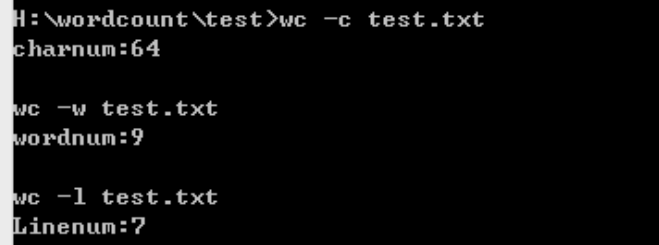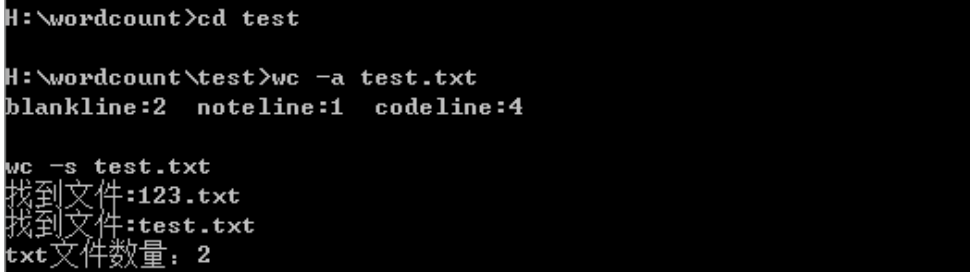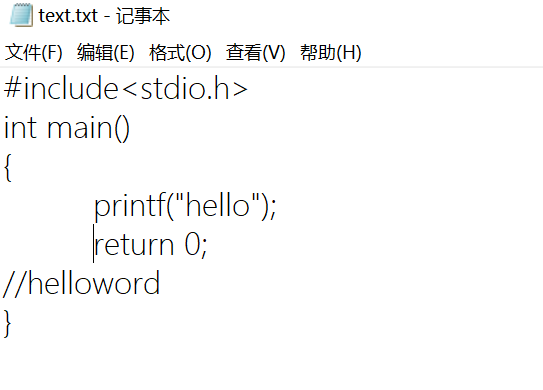## （1）互审代码情况

1.循环的局部变量使用了一些简单字母，不容易看出变量的含义；
2.注释不够详细，比如没有注释解释一些循环的作用，一些变量的意义。

## （2）静态代码检查情况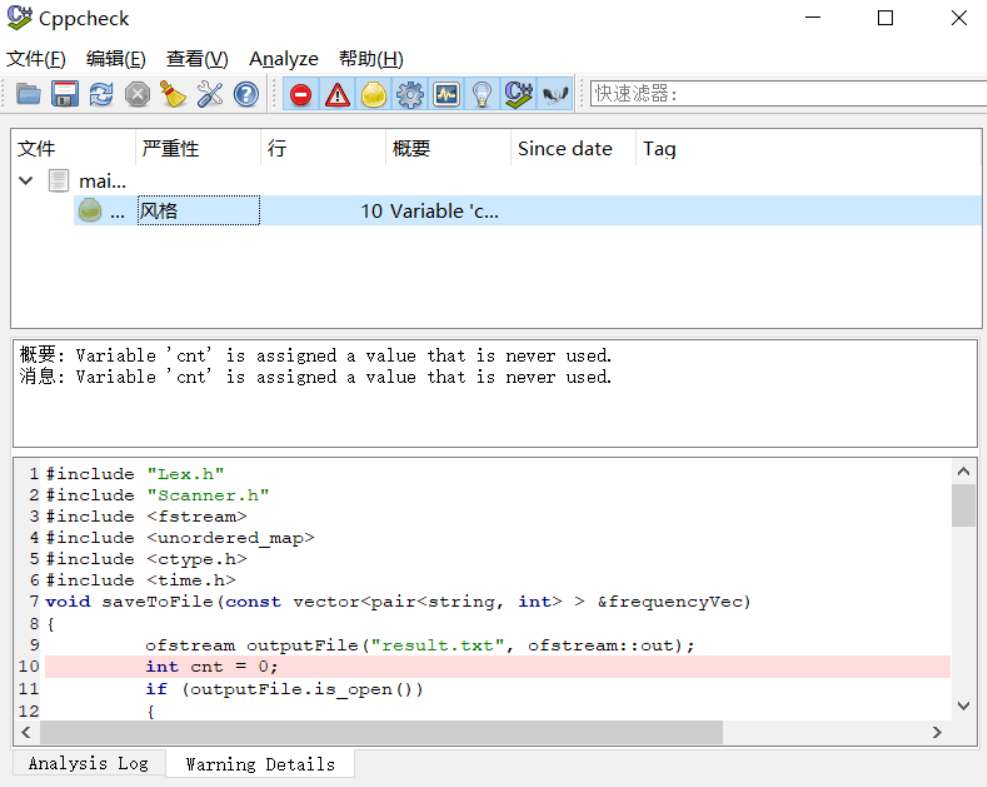## （3）单元测试情况

case1:文本中全部是常见字符；
case2:文本中全部是常见字符和非常见字符；
case3:文本中是常见字符和少量英文字母；
case4:文本中全部是数字；
case5:文本中是数字和少量英文字母；
case6:文本中包含Let's和Let''s；
······

## 单元测试运行截图：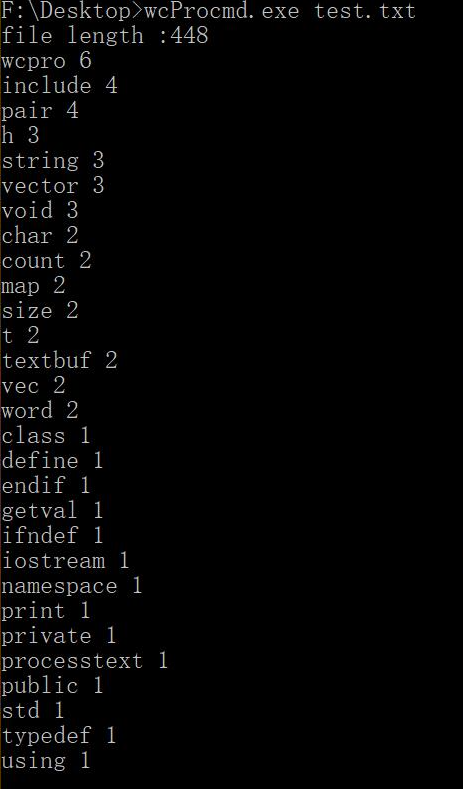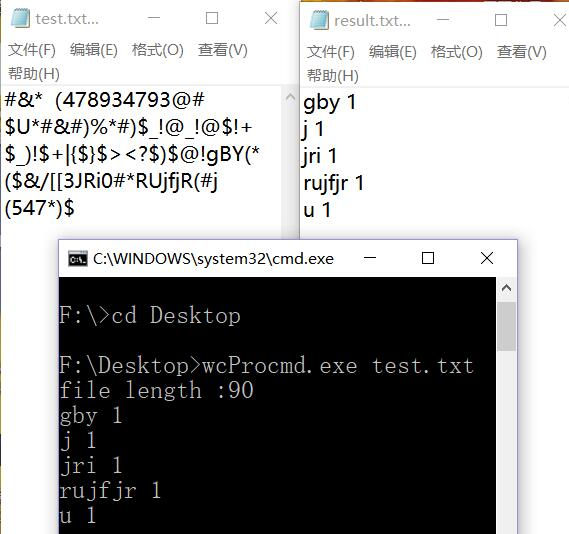## 参考文件：

### 总结：

posted @ 2018-10-21 13:19  俊熙是我真名啊  阅读(203)  评论(0编辑  收藏  举报
Live2D